# 【数学建模学习笔记【集训十天】之第六天】

### 数模学习目录

• Matplotlib 学习
• Matplotlib简介
• Matplotlib 散点图
• 运行效果如下：
• Matplotlib Pyplot
• 运行效果如下：
• 关于plot()
• 运行效果如下：
• 绘制任意数量的点
• 运行效果如下：
• 不指定 x 轴上的点,看看效果
• 运行效果如下：
• 再看看y更多值的效果
• 运行效果如下：
• 绘制正余弦图：
• 运行效果如下：
• 两个数据集
• 运行效果如下：
• Matplotlib 绘图标记
• 实心圆标记：
• 运行效果如下:
• 用 * 标记
• 运行效果如下：
• 用下箭头标记
• 运行效果如下：
• Matplotlib 折线图
• 运行效果如下：
• fmt 参数
• 运行效果如下：
• 标记大小与颜色
• 设置标记大小:
• 运行效果如下：
• 设置标记外边框颜色：
• 运行效果如下：
• 设置标记内部颜色：
• 运行效果如下：
• Matplotlib 条形图
• 运行效果如下：
• 总结
• 上一章链接：[【数学建模学习笔记【集训十天】之第五天】](https://blog.csdn.net/m0_66318554/article/details/125556546)
• 每日一言：
• 持续更新中

• # Matplotlib 学习

## Matplotlib简介

``````# -*- coding = utf-8 -*-
# @Time : 2022/7/2 10:42
# @Author : lxw_pro
# @File : Matplotlib 学习.py
# @Software : PyCharm

# Matplotlib简介：
'''
使用 Matplotlib 在 Python 中创建图表【Matplotlib 是用于创建图表的 Python 库】
Matplotlib 是一个非常强大的 Python 画图工具，我们可以使用该工具将很多数据通过图表的形式更直观的呈现出来。
它能让使用者很轻松地将数据图形化，并且提供多样化的输出格式【可创建散点图，折线图，条形图和饼图等】
Matplotlib 可以用来绘制各种静态，动态，交互式的图表。

注：在使用之前需在终端安装Matplotlib
安装命令为：pip install matplotlib

Matplotlib应用：
Matplotlib 通常与 NumPy 和 SciPy（Scientific Python）一起使用， 这种组合广泛用于替代 MatLab，
是一个强大的科学计算环境，有助于我们通过 Python 学习数据科学或者机器学习

'''

# 查看版本
import matplotlib

print(matplotlib.__version__)

``````

## Matplotlib 散点图

``````# Matplotlib 散点图
# 散点图是一种图形或数学图，使用笛卡尔坐标显示一组数据的两个变量的值

# 使用时，我们可以使用 import 导入 pyplot 库，并设置一个别名 plt
import matplotlib.pyplot as plt

x_hz = [i for i in range(10)]                   # 时间数据
y_zz = [6, 14, 21, 36, 45, 52, 66, 72, 88, 92]  # 价格数据

plt.title('price for 10 years')                 # 设置图表标题
plt.scatter(x_hz, y_zz, color='blue', marker='x', label='item 1')   # scatter()功能绘制散点图

plt.xlabel('year')      # 设置x轴标签
plt.ylabel('price')     # 设置y轴标签

plt.grid(True)          # grid()功能显示网格
plt.legend()            # legend()功能在轴上放置图例

plt.show()              # 显示图表

``````

### 运行效果如下：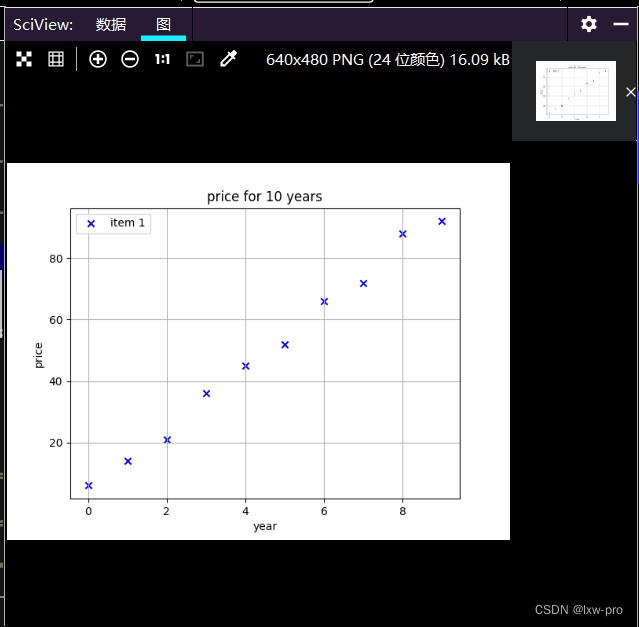### Matplotlib Pyplot

``````# Matplotlib Pyplot
'''
Pyplot 是 Matplotlib 的子库，提供了和 MATLAB 类似的绘图 API。

Pyplot 是常用的绘图模块，能很方便让用户绘制 2D 图表。

Pyplot 包含一系列绘图函数的相关函数，每个函数会对当前的图像进行一些修改

'''

import matplotlib.pyplot as plt
import numpy as np

x_d = np.array([0, 8])
y_d = np.array([0, 120])

plt.plot(x_d, y_d)  # plot() 函数是绘制二维图形的最基本函数
plt.show()

``````

#### 运行效果如下：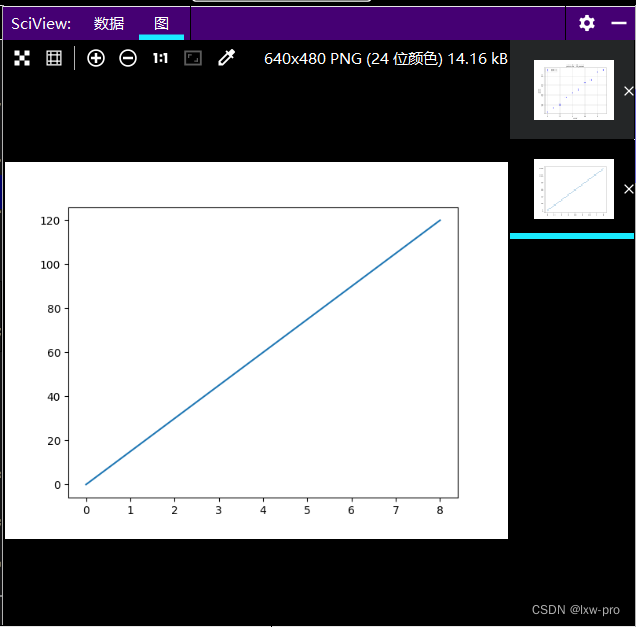### 关于plot()

``````# 关于plot()
'''
plot() 用于画图它可以绘制点和线，语法格式如下：

# 画单条线
plot([x], y, [fmt], *, data=None, **kwargs)
# 画多条线
plot([x], y, [fmt], [x2], y2, [fmt2], ..., **kwargs)
参数说明：

x, y：点或线的节点，x 为 x 轴数据，y 为 y 轴数据，数据可以列表或数组。
fmt：可选，定义基本格式（如颜色、标记和线条样式）。
**kwargs：可选，用在二维平面图上，设置指定属性，如标签，线的宽度等。

颜色字符：'b' 蓝色，'m' 洋红色，'g' 绿色，'y' 黄色，'r' 红色，'k' 黑色，'w' 白色，'c' 青绿色，'#008000' RGB 颜色符串。多条曲线不指定颜色时，会自动选择不同颜色。

线型参数：'‐' 实线，'‐‐' 破折线，'‐.' 点划线，':' 虚线。

标记字符：'.' 点标记，',' 像素标记(极小点)，'o' 实心圈标记，'v' 倒三角标记，'^' 上三角标记，'>' 右三角标记，'<' 左三角标记...等等

'''

# 可使用 o 参数，表示一个实心圈的标记
import matplotlib.pyplot as plt
import numpy as np

x_p = np.array([0, 6])
y_p = np.array([3, 9])

plt.plot(x_p, y_p, 'o')
plt.show()

``````

#### 运行效果如下：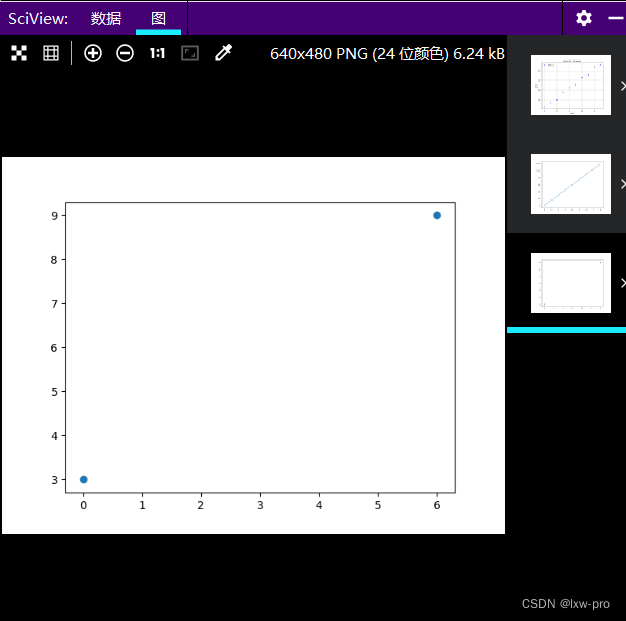### 绘制任意数量的点

``````# 绘制任意数量的点
import matplotlib.pyplot as plt
import numpy as np

x_dp = np.array([1, 3, 5, 7, 9])
y_dp = np.array([8, 4, 2, 6, 0])

plt.plot(x_dp, y_dp)
plt.show()

``````

#### 运行效果如下：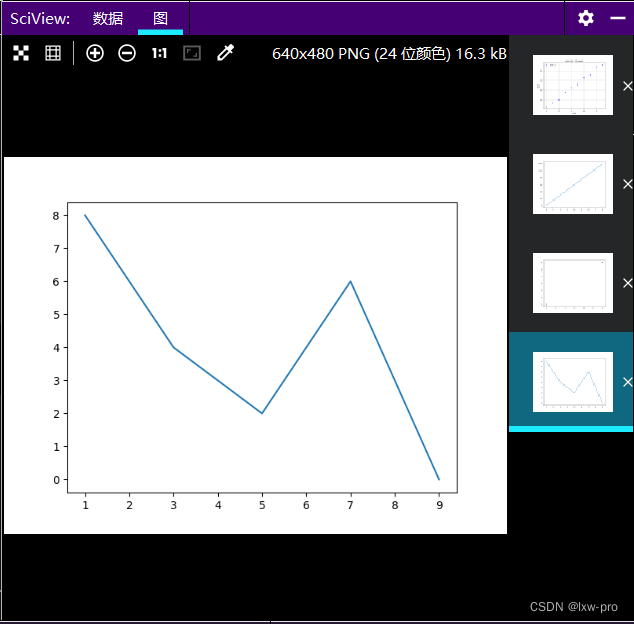### 不指定 x 轴上的点,看看效果

``````# 不指定 x 轴上的点,看看效果
import matplotlib.pyplot as plt
import numpy as np

yd = np.array([2, 9])

plt.plot(yd)
plt.show()
``````

#### 运行效果如下：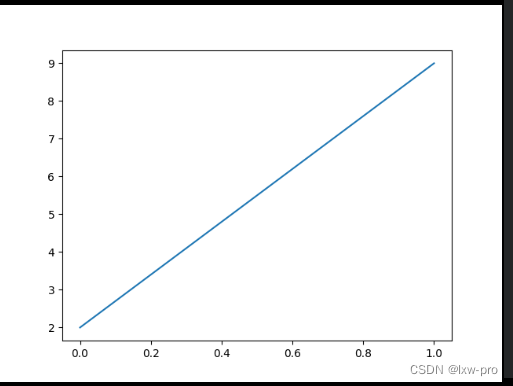### 再看看y更多值的效果

``````# 再看看y更多值的效果
import matplotlib.pyplot as plt
import numpy as np

yd2 = np.array([2, 9, 1, 8, 3, 8])

plt.plot(yd2)
plt.show()

``````

#### 运行效果如下：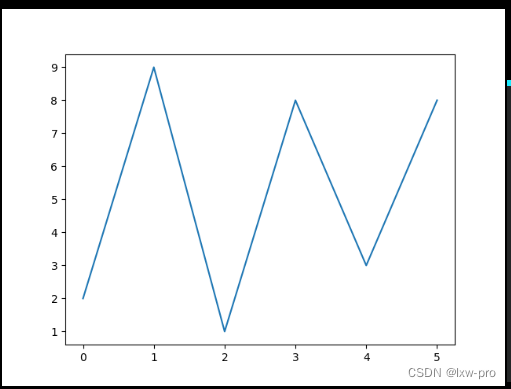## 绘制正余弦图：

``````# 绘制正余弦图：
import matplotlib.pyplot as plt
import numpy as np

x = np.arange(0, 4*np.pi, 0.1)
y = np.sin(x)
z = np.cos(x)

plt.plot(x, y, x, z)
plt.show()

``````

### 运行效果如下：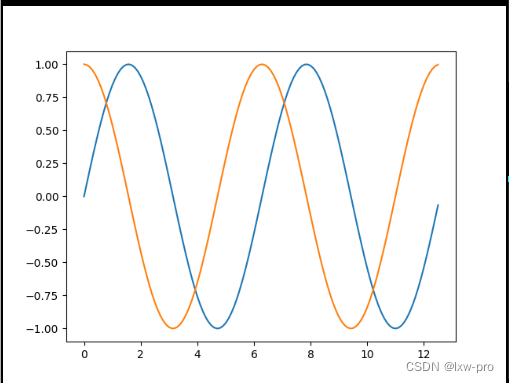## 两个数据集

``````# 两个数据集
import matplotlib.pyplot as plt

x_d1 = [i for i in range(1, 11)]
y_d1 = [2, 32, 41, 6, 15, 34, 34, 23, 12, 9]

x_d2 = [i for i in range(1, 11)]
y_d2 = [4, 12, 43, 21, 52, 32, 12, 43, 8, 20]

plt.title('Prices for 10 years')

plt.scatter(x_d1, y_d1, color='darkblue', marker='x', label='item 1')
plt.scatter(x_d2, y_d2, color='darkred', marker='x', label='item 2')

plt.xlabel('Year')
plt.ylabel('Price')

plt.grid(True)
plt.legend()

plt.show()

``````

### 运行效果如下：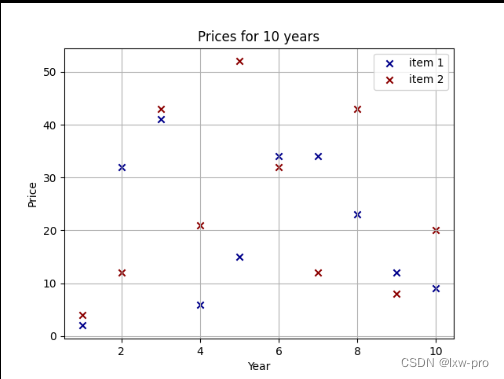## Matplotlib 绘图标记

### 实心圆标记：

``````# Matplotlib 绘图标记
# 绘图过程可使用 plot() 方法的 marker 参数来定义标记

# 实心圆标记：
import matplotlib.pyplot as plt
import numpy as np

yp = np.array([1, 3, 1, 4, 1, 5, 9, 5, 2, 1])

plt.plot(yp, marker='o')
plt.show()

``````

#### 运行效果如下: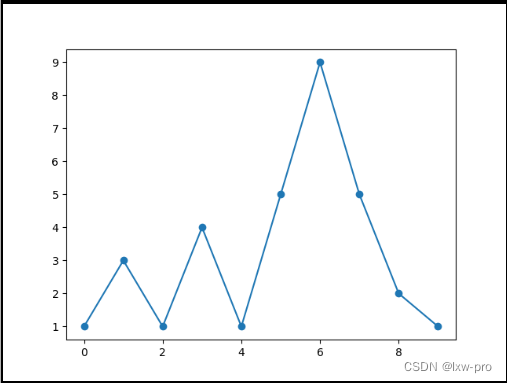### 用 * 标记

``````# 用 * 标记
import matplotlib.pyplot as plt
import numpy as np

yp2 = np.array([2, 3, 2, 43, 23, 12, 3, 4, 12, 43, 12])

plt.plot(yp, marker='*')
plt.show()

``````

#### 运行效果如下：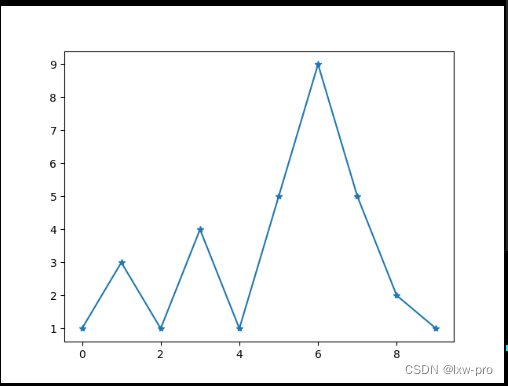### 用下箭头标记

``````# 用下箭头标记
import matplotlib.pyplot as plt
import matplotlib.markers

plt.plot([1, 3, 5], marker=matplotlib.markers.CARETUPBASE)
plt.show()

``````

#### 运行效果如下：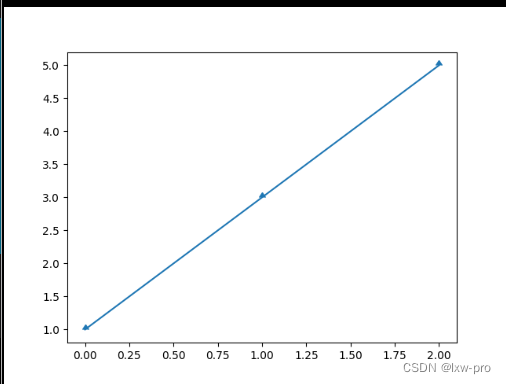## Matplotlib 折线图

``````# Matplotlib 折线图
# 折线图是一种显示图表的图表，该信息显示为一系列数据点，这些数据点通过直线段相连，称为标记

# 正弦波折线图:
import matplotlib.pyplot as plt
import numpy as np

t = np.arange(0.0, 4.0, 0.01)   # arange()函数返回给定间隔内的均匀间隔的值列表
s = np.sin(2.5*np.pi*t)

# plot()功能绘制折线图
plt.plot(t, s)

plt.title('Wave')

plt.xlabel('Time')
plt.ylabel('Vol')

plt.grid(True)
plt.show()

``````

### 运行效果如下：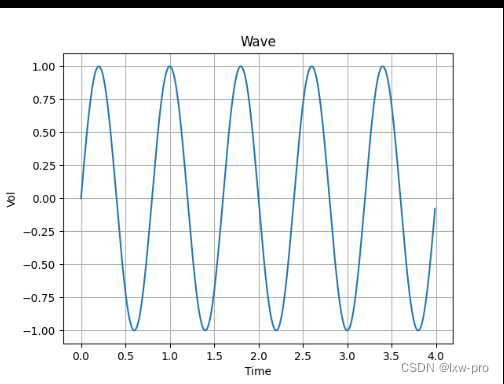## fmt 参数

``````# fmt参数
'''
fmt 参数
fmt 参数定义了基本格式，如标记、线条样式和颜色。

fmt = '[marker][line][color]'
如   o:r【o 表示实心圆标记，: 表示虚线，r 表示颜色为红色】

:  代表虚线        -  代表实线
-- 代表破折线      -. 代表点划线

除了黑色是用k表示，其他颜色均用各自英文首字母表示

'''
import matplotlib.pyplot as plt
import numpy as np

ft = np.array([3, 9, 2, 6])
plt.plot(ft, 'o:r')

plt.show()

``````

### 运行效果如下：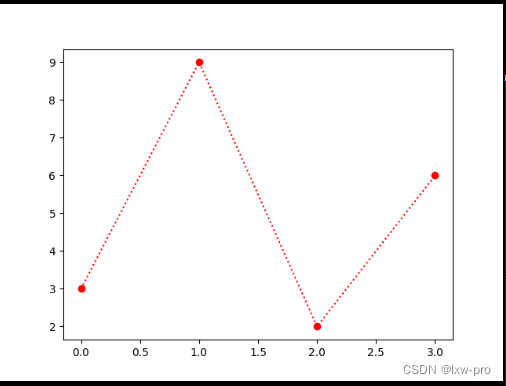## 标记大小与颜色

### 设置标记大小:

``````# 标记大小与颜色
'''
标记大小与颜色
我们可以自定义标记的大小与颜色，使用的参数分别是：
markersize，简写为 ms：定义标记的大小。
markerfacecolor，简写为 mfc：定义标记内部的颜色。
markeredgecolor，简写为 mec：定义标记边框的颜色。

'''

# 设置标记大小:
import matplotlib.pyplot as plt
import numpy as np

dx = np.array([2, 32, 1, 43, 12, 12, 3])
plt.plot(dx, marker='o', ms=20)

plt.show()

``````

#### 运行效果如下：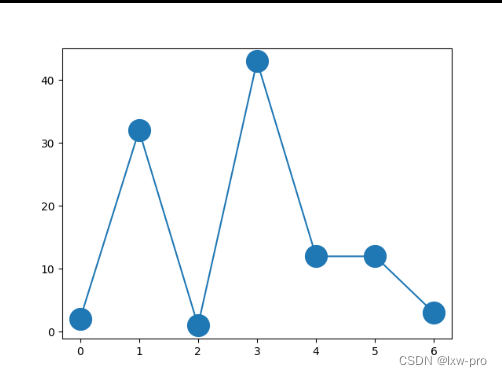### 设置标记外边框颜色：

``````# 设置标记外边框颜色：
import numpy as np
import matplotlib.pyplot as plt

yys = np.array([2, 1, 43, 1, 2, 12, 1])
plt.plot(yys, marker='*', ms=20, mec='g')

plt.show()

``````

#### 运行效果如下：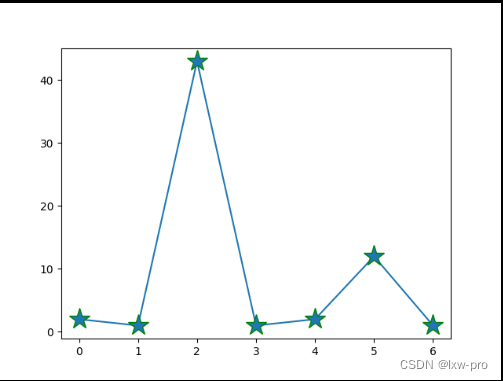### 设置标记内部颜色：

``````# 设置标记内部颜色：
import matplotlib.pyplot as plt
import numpy as np

nys = np.array([2,3, 32, 12, 43, 12, 9])
plt.plot(nys, marker='o', ms=20, mfc='r')

plt.show()

``````

#### 运行效果如下：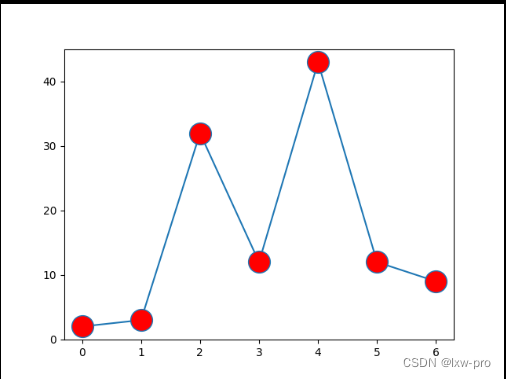## Matplotlib 条形图

``````# Matplotlib 条形图
# 条形图显示带有矩形条的分组数据，其长度与它们代表的值成比例【条形图可以垂直或水平绘制】
import matplotlib.pyplot as plt
import matplotlib.style as sty

sty.use('ggplot')

x = [i for i in range(1, 4)]
y = [12, 8, 4]

fuck, ax = plt.subplots()       # subplots()函数返回图形和轴对象

ax.bar(x, y, align='center')    # bar()功能生成条形图

ax.set_title('Olympic Gold for London')
ax.set_ylabel('Gold medals')
ax.set_xlabel('Countries')

ax.set_xticks(x)
ax.set_xticklabels(('China', 'UK', 'USA'))

plt.show()

``````

### 运行效果如下：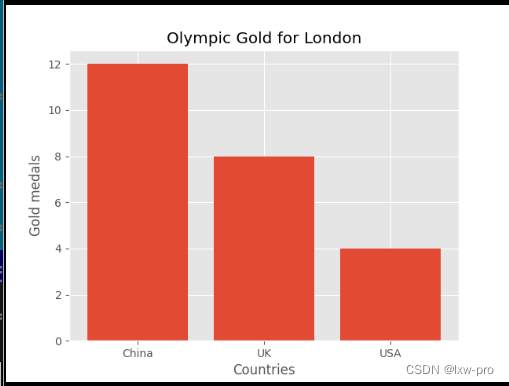### 总结

我写的之后才知道，光是想写是不够的，要学的东西还有很多很多，不过这些暂时都是次要的，主要的还是享受当前的学习，感受当前学习中的乐趣。

## 上一章链接：【数学建模学习笔记【集训十天】之第五天】

### 每日一言：

• 我对赢没兴趣，只是不想输而已！！！

• #### 持续更新中来源：lxw-pro